# Python operator module with examples

To perform operations using methods instead of operators in a Python program, we have an "operator" module that provides a lot of methods to perform the operations. This article deals with these methods available in Python's operator module:

The details, like a brief description and example of all methods, are given below, one by one. Let's have a look at all these to learn everything about the operator module's methods. To use any of the above methods, we must import the operator module. And all the methods must be used along with the operator, as shown in the syntax given below:

```import operator

operator.method_name()```

The operator module's add() method works in the same way as the + operator. That is, this method is used for addition. To use the add() method, we can proceed as follows:

`res = operator.add(a, b)`

The above statement indicates that the addition result of a and b gets initialized to the res variable. Don't forget to import the operator module before using its methods, like add(). To import the operator module, use the following statement:

`import operator`

The statement, "res = operator.add(a, b)" is equivalent to:

`res = a + b`

Here is an example program that implements the add() method of the operator module:

```import operator

a, b = 10, 20
print(res)```

Here is a snapshot of the output produced by the above program:## The sub() method

Just like the add() method, the sub() method is used for subtraction. Here is an example program that uses the sub() method of the operator module:

```import operator

a, b = 6, 4
res = operator.sub(a, b)
print(res)```

The output will be 2.

## The mul() method

The mul() method works the same as *, used for multiplication. Here's an example program that makes use of the mul() method:

```import operator

a, b = 6, 4
res = operator.mul(a, b)
print(res)```

24 is the output this program generates.

## The truediv() method

The truediv() method works the same as /, used for division. The example given below uses the truediv() method:

```import operator

a, b = 6, 4
res = operator.truediv(a, b)
print(res)```

The output produced by this program will be 1.5, that is, the true or exact division result.

## The floordiv() method

The floordiv() method of the operator module works the same as //, which is also used for division. But the result that comes out after dividing two numbers using floordiv() will not be the exact division result. Rather, it will be the nearest integer value that is less than or equal to the result. Here is an example program for floordiv():

```import operator

a, b = 6, 4
res = operator.floordiv(a, b)
print(res)```

The output produced by the above program is 1.

## The mod() method

The mod() method works the same as %, used to find modulo. The example program that uses the mod() method of the operator module is given below:

```import operator

a, b = 6, 4
res = operator.mod(a, b)
print(res)```

The output produced by the above program will be 2, which shows the remainder value when dividing 6 by 4.

## The pow() method

The pow() method of operator module works same as **, used for exponentiation. That is a ** b interpreted as ab. Here's an example of a program that makes use of the pow() method:

```import operator

a, b = 2, 5
res = operator.pow(a, b)
print(res)```

The output this program generates is 32. Since operator.pow(a, b) evaluates to ab, and this again evaluates to 25, we get 32.

## The eq() method

The eq() method of the operator module works the same as ==, used to check for equality. That is, whether any two variables, values, or operands are equal or not. Here's an example of how to use the eq() method:

```import operator

a, b = 6, 5
res = operator.eq(a, b)
print(res)```

The output produced by the above program is false since the value of a is not equal to the value of b. Here is another program:

```import operator

print("Enter first Input: ", end="")
a = input()
print("Enter second Input: ", end="")
b = input()
if operator.eq(a, b):
print("\nBoth are equal")
else:
print("\nBoth are not equal")```

Here is a sample run with "codescracker" as both the first and second input from the user:And here is another sample run with user input 8 as both first and second input: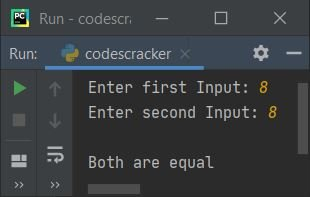That is, because the expression operator.eq(8, 8) evaluates to true, the program flow enters the if's block and executes the print statement, which prints "Both are equal."

## The ne() method

The ne() method works the same as "!=", which is used to check whether any two variables or directly assigned values are equal to each other or not. That is, using this method, if both variables are not equal to each other, then it returns true, otherwise false gets returned. Here's an example of a program that makes use of the ne() (not equal) method:

```import operator

a, b = 6, 5
res = operator.ne(a, b)
print(res)```

This program produces "true" as an output, since 6 is not equal to 5.

## The gt() method

The gt() method of the operator module works the same as > and is used to check whether the first argument passed is greater than the second argument passed or not. True is returned if the first one is greater than the second; otherwise, false is returned. Here's an example of a program that makes use of the gt() method:

```import operator

a, b = 6, 5
res = operator.gt(a, b)
print(res)```

The output will be true since a's value is greater than b's value. Here is another example that shows the famous use of the gt() method:

```import operator

print("Enter first Number: ", end="")
num_one = int(input())
print("Enter second Number: ", end="")
num_two = int(input())
if operator.gt(num_one, num_two):
print(num_one, "is greater than", num_two)
else:
print(num_one, "is less than", num_two)```

Here's a sample run with 20 as the first number and 10 as the second: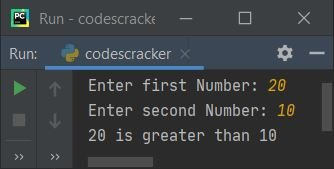## The lt() method

The lt() method works the same as "<" and is used to check whether the value of the first argument is less than the value of the second argument (passed to the method) or not. Here's an example of how to use the operator module's lt() method:

```import operator

a, b = 6, 5
res = operator.lt(a, b)
print(res)```

Because a (6) is greater than b (5), the output is false.

## The ge() method

The ge() method of the operator module works the same as the ">=" operator and is used to check whether the first argument is greater than or equal to the second argument passed to the method or not. It returns either true or false. Here is an example using the ge() (greater than or equal to) method:

```import operator

a, b = 6, 5
res = operator.ge(a, b)
print(res)```

Because a (6) is greater than b (5), the output is true.

## The le() method

The le() method works the same as the "<=" operator and is used to check whether the first argument passed is less than or equal to the second argument passed to the method or not. It returns either true or false. The example program that uses the le() method is given below:

```import operator

a, b = 6, 5
res = operator.le(a, b)
print(res)```

produces false output.

## The and_() method

The operator module's and_() method works in the same way as the Bitwise AND operator. Bitwise Operators with Example contains all of the information you need about bitwise operators. Here's an example of the and_() method in action:

```import operator

a, b = 60, 13
res = operator.and_(a, b)
print(res)```

The output produced by the above program will be 12. To learn how the calculation on the bitwise AND operator gets performed, refer to the separate article provided just above the program. But for now, here is another example program that may remind you about the operator:

```import operator

a, b = 1, 0
print(a, "&", b, "=", operator.and_(a, b))
a, b = 0, 1
print(a, "&", b, "=", operator.and_(a, b))
a, b = 0, 0
print(a, "&", b, "=", operator.and_(a, b))
a, b = 1, 1
print(a, "&", b, "=", operator.and_(a, b))```

The screenshot provided below displays the output this Python program generated:## The or_() method

The or_() method is equivalent to the | operator. Here is an example using this method:

```import operator

a, b = 60, 13
res = operator.or_(a, b)
print(res)```

produces 61 outputs. That is, while taking the bitwise OR between the binary equivalents of both values, say 60 and 13, we'll get another binary value, which is of course equal to 61.

## The xor() method

The xor() method works the same as the "^" operator. Here is an example program that uses this method:

```import operator

a, b = 60, 13
res = operator.xor(a, b)
print(res)```

produces "49" on the output console.

## The invert() method

The invert() method is equivalent to "˜"
This method is used to invert the binary value or binary equivalent of a value. Unlike the above methods, this method works on a single argument. Here's an example of how to use the invert() method:

```import operator

a = 5
print(operator.invert(a))```

produces -6 as an output.

## The lshift() method

The lshift() function is equivalent to the "<<" operator. Here's an example of how to use the operator module's lshift() method:

```import operator

a, b = 14, 1
print(operator.lshift(a, b))```

Because shifting the binary equivalent of 14 to the left by one position yields a binary value equal to 28 (in decimal), we get 28 as an output.

## The rshift() method

The rshift() works same as ">>" operator. Here's an example of the rshift method in action:

```import operator

a, b = 14, 1
print(operator.rshift(a, b))```

Because shifting the binary equivalent value (0000 1110) of 14 to 1 position left yields a binary value (0000 0111) equal to 7 in decimal, we get 7.

## The is_() method

The is_() method of the operator module works the same as the "is" identity operator. Here's an example of the is_() method in action:

```import operator

a, b, = 10, 10
print(operator.is_(a, b))

a, b = "codes", "cracker"
print(operator.is_(a, b))

a, b = "codescracker", "codescracker"
print(operator.is_(a, b))```

The snapshot given below shows the sample output produced by the above program: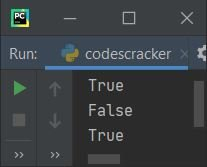## The is_not() method

The is_not() function works in the same way as the "is not" operator. Here is an example using this method:

```import operator

a, b, = 10, 10
print(operator.is_not(a, b))

a, b = "codes", "cracker"
print(operator.is_not(a, b))

a, b = "codescracker", "codescracker"
print(operator.is_not(a, b))```

The output produced by this program looks like this: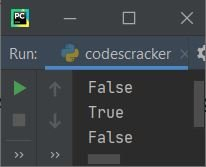## The contains() method

The contains() method of the operator module works the same as the "in" operator; it checks whether an object is a member of any list, string, or tuple or not. Here's an example of the contains() method in action:

```import operator

nums = [1, 2, 3, 4, 5]
val = 3

res = operator.contains(nums, val)
print(res)

print(operator.contains(nums, 6))```

Here is a snapshot of the output produced by the above Python program: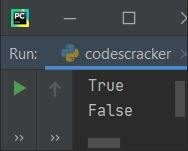The program given below shows the major and most popular use of the contains() method in Python programming, which is to search for an element:

```import operator

nums = [1, 2, 3, 4, 5]

print("Enter an element to search: ")
val = int(input())

if operator.contains(nums, val):
print("\nIt is available in the list")
else:

Here is its sample run with user input of 10:## The concat() method

The concat() method of the operator module works the same as + while concatenating two strings. This method takes two arguments, and both arguments must be of the string type. Here is an example:

```import operator

a = "codes"
b = "cracker"
res = operator.concat(a, b)
print(res)```

The output produced by the above program will be "codescracker."

## The getitem() method

The getitem() method is used to get an element. The following code or statement:

`val = operator.getitem(nums, index_number)`

is similar to:

`val = nums[index_number]`

Here's an example program that makes use of the operator module's getitem() method:

```import operator

nums = [10, 20, 30, 40, 50]
print("Get element with Index Number: ", end="")
index_number = int(input())

val = operator.getitem(nums, index_number)
print("\nThe value at index", index_number, "is", val)```

Here is its sample run with user input, using 3 as the index number to get the element at this index number: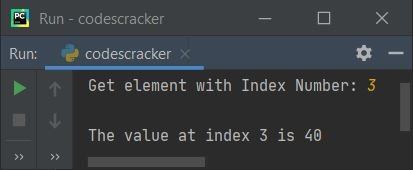The same method, getitem(), can also be used to get multiple items. To accomplish this, use a method like "getitem(nums, slice(i, j))" to slice all items from index i to j from nums. The slicing is performed in such a way that the first index (i) is included whereas the second index (j) is excluded. That is, "getitem(nums, slice(1, 3))" sliced elements at the first and second indexes only, but not at the third index. Here is an example:

```import operator

nums = [10, 20, 30, 40, 50]
print(operator.getitem(nums,slice(1, 3)))```

The output produced by the above program will be [20, 30].

## The setitem() method

The setitem() method, which is also called "indexed assignment," is used to replace an item in a list. For example, consider the following statement:

`operator.setitem(nums, index_number, new_value)`

is similar to:

`nums[index_number] = new_value`

Here's an example program that makes use of the operator module's setitem() method:

```import operator

nums = [10, 20, 30, 40, 50]
operator.setitem(nums, 2, 3)
print(nums)```

Here is its sample output: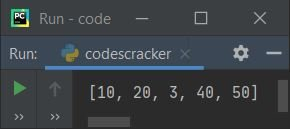The setitem() method can also be used to assign multiple values at a time. This can be used in conjunction with slice assignment. To do this, we need to use the setitem() method as setitem(seq, slice(i, j), values), which is similar to:

`seq[i:j] = values`

Here is an example program:

```import operator

nums = [10, 20]
operator.setitem(nums, slice(2, 5), [30, 40, 50])
print(nums)```

Because the setitem() method inserts three new elements, the output is [10, 20, 30, 40, 50].

## The delitem() method

The delitem() method of the operator module, also known as indexed deletion, is used to delete an element. The delitem() method is used in the following code:

`operator.delitem(nums, index_number)`

is similar to:

`del nums[index_number]`

Here is an example program that uses the delitem() method:

```import operator

nums = [10, 20, 30, 40, 50]
operator.delitem(nums, 2)
print(nums)```

After executing the above program, we'll get the following output:Just like setitem(), the delitem() method can also be used to delete multiple elements at a time using slice deletion. This can be done using the same method in a way like "delitem(seq, slice(i, j))," which works similarly to:

`del seq[i:j]`

Here is an example program that uses the delitem() method to delete multiple items:

```import operator

nums = [10, 20, 30, 40, 50]
operator.delitem(nums, slice(2, 5))
print(nums)```

Produces the following output: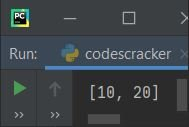Python Online Test

« Previous Tutorial Next Tutorial »